Potential difference.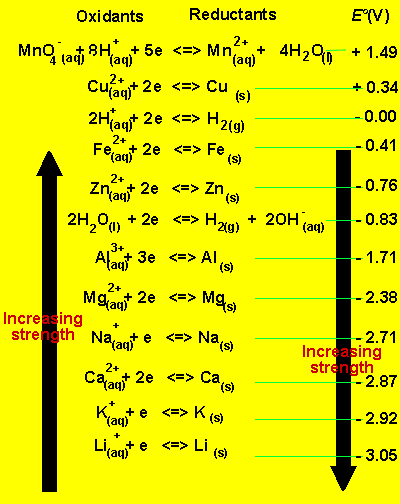We can predict the voltage of a cell by using the table on the left of standard half cell potentials. These half cell potentials were measured against the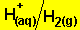half cell. The hydrogen half cell was arbitrarily assigned a value of 0V. When the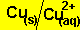half cell is connected to thehalf cell a potential difference of 0.34V exists. The copper half cell is 0.34V greater in potential than the hydrogen half cell. Similarly when the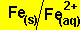half cell is connected to the hydrogen half cell a difference of 0.41V in favour of the hydrogen half cell exists. In developing this half cell potential table we can now start to predict the potential difference of a given cell.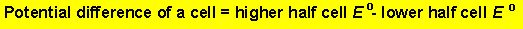Example 1) Using the table above, predict the cell potential difference when the two half cells below are connected.and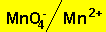. Step 1 Potential difference = highest - lowest Step 2 Using the table above write down the values potential difference = 1.49 - 0.34 =1.15V Example 2) Using the table above, predict the cell potential difference when the two half cells below are connected.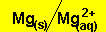andStep 1 Potential difference = highest - lowest Step 2 Using the table above write down the values potential difference = 0.34 - -2.38 = 0.34 + 2.38 = 2.72V Exercises

Home